4 Magnetic anisotropy and domains

## Chapter 4Magnetic anisotropy and domains

Rocks often contain assemblages of ferromagnetic minerals dispersed within a matrix of diamagnetic and paramagnetic minerals. In later chapters we will be concerned with the magnetization of these assemblages, but here we continue our investigation of the behavior of individual particles. In Chapter 3 we learned that in some crystals electronic spins work in concert to create a spontaneous magnetization that remains in the absence of an external field. The basis of paleomagnetism is that these ferromagnetic particles carry the record of ancient magnetic fields. What allows the magnetic moments to come into equilibrium with the geomagnetic field and then what fixes that equilibrium magnetization into the rock so that we may measure it millions or even billions of years later? We will begin to answer these questions over the next few chapters.

We will start with the second part of the question: what fixes magnetizations in particular directions? A basic principle is that ferromagnetic particles have various contributions to the magnetic energy which controls their magnetization. No matter how simple or complex the combination of energies may become, the grain will seek the configuration of magnetization which minimizes its total energy. The short answer to our question is that certain directions within magnetic crystals are at lower energy than others. To shift the magnetization from one “easy” direction to another requires energy. If the barrier is high enough, the particle will stay magnetized in the same direction for very long periods of time – say billions of years. In this chapter we will address the causes and some of the consequences of these energy barriers for the magnetization of rocks. Note that in this chapter we will be dealing primarily with energy densities (volume normalized energies), as opposed to energy and will distinguish the two by the convention that energies are given with the symbol E and energy densities with ϵ.

In Chapter 6, we will discuss the behavior of common magnetic minerals, but to develop the general theory, it is easiest to focus on a single mineral. We choose here the most common one, magnetite. It has a simple, cubic structure and has been the subject intensive study. However, we will occasionally introduce concepts appropriate for other magnetic minerals where appropriate.

The simplest permanently magnetized particles are quasi-uniformly magnetized. These so-called single domain (SD) particles have spins that act in concert, staying as parallel (or anti-parallel) as possible. As particles get larger, the external energy can be minimized by allowing neighboring spins to diverge somewhat from strict parallelism; these particles are referred to as pseudo-single domain or PSD. Eventually, the spins organize themselves into regions with quasi-uniform magnetization (magnetic domains) separated by domain walls and are called multi-domain (MD) particles. These more complicated spin structures are very difficult to model and most paleomagnetic theory is based on the single domain approximation. Therefore we begin with a discussion of the energies of uniformly magnetized (single-domain) particles.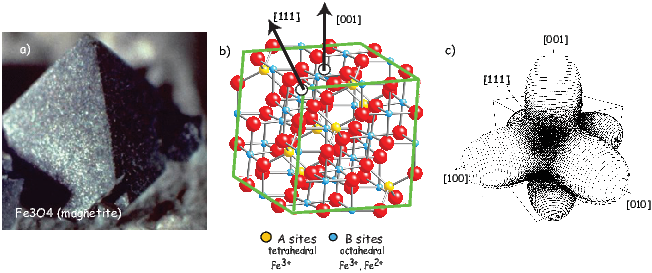Figure 4.1: a) A magnetite octahedron. [Photo of Lou Perloff in The Photo-Atlas of Minerals.] b) Internal crystal structure. Directions of the body diagonal ( direction) and orthogonal to the cubic faces ( direction) are shown as arrows. Big red dots are the oxygen anions. The blue dots are iron cations in octahedral coordination and the yellow dots are in tetrahedral coordination. Fe3+ sits on the A sites and Fe2+ and Fe3+ sit on the B sites. c) Magnetocrystalline anisotropy energy as a function of direction within a magnetite crystal at room temperature. The easiest direction to magnetize (the direction with the lowest energy – note dimples in energy surface) is along the body diagonal (the  direction). [Figure from Williams and Dunlop, 1995.]

### 4.1 The magnetic energy of particles

#### 4.1.1 Exchange energy

We learned in Chapter 3 that some crystalline states are capable of ferromagnetic behavior because of quantum mechanical considerations. Electrons in neighboring orbitals in certain crystals “know” about each other’s spin states. In order to avoid sharing the same orbital with the same spin (hence having the same quantum numbers – not allowed by Pauli’s exclusion principle), electronic spins in such crystals act in a coordinated fashion. They will be either aligned parallel or antiparallel according to the details of the interaction. This exchange energy density (ϵe) is the source of spontaneous magnetization and is given for a pair of spins by: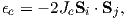where Je is the exchange integral and Si and Sj are spin vectors. Depending on the details of the crystal structure (which determines the size and sign of the exchange integral), exchange energy is at a minimum when electronic spins are aligned parallel or anti-parallel.

We define here a parameter that we will use later: the exchange constant A = JeS2∕a where a is the interatomic spacing. A = 1.33 x 10-11 Jm-1 for magnetite, a common magnetic mineral.

Recalling the discussion in Chapter 3, while s orbitals which are spherical, the 3d electronic orbitals “poke” in certain directions. Hence spins in some directions within crystals will be easier to coordinate than in others. We can illustrate this using the example of magnetite, a common magnetic mineral (Figure 4.1a). Magnetite octahedra (Figure 4.1a), when viewed at the atomic level (Figure 4.1b) are composed of one ferrous (Fe2+) cation, two ferric (Fe3+) cations and four O2- anions. Each oxygen anion shares an electron with two neighboring cations in a covalent bond.

In Chapter 3 we mentioned that in some crystals, spins are aligned anti-parallel, yet there is still a net magnetization, a phenomenon we called ferrimagnetism. This can arise from the fact that not all cations have the same number of unpaired spins. Magnetite, with its ferrous (4 mb) and ferric (5 mb) states is a good example. There are three iron cations in a magnetite crystal giving a total of 14 mb to play with. Magnetite is very magnetic, but not that magnetic! From Figure 4.1b we see that the ferric ions all sit on the tetrahedral (A) lattice sites and there are equal numbers of ferrous and ferric ions sitting on the octahedral (B) lattice sites. The unpaired spins of the cations in the A and B lattice sites are aligned anti-parallel to one another because of superexchange (Chapter 3) so we have 9 mb on the B sites minus 5 mb on the A sites for a total of 4 mb per unit cell of magnetite.

#### 4.1.2 Magnetic moments and external fields

We know from experience that there are energies associated with magnetic fields. Just as a mass has a potential energy when it is placed in the gravitational field of another mass, a magnetic moment has an energy when it is placed in a magnetic field. We have seen this energy briefly in Sections 1.4 and Equation 3.4. This energy has many names (magnetic energy, magnetostatic energy, Zeeman energy, etc.). Here we will work with the volume normalized magnetostatic interaction energy density (ϵm). This energy density essentially represents the interaction between the magnetic lines of flux and the magnetic moments of the electronic spins. It is energy that aligns magnetic compass needles with the ambient magnetic field. We find the volume normalized form (in units of Jm-3) by substituting |M| = |m|v1 2 (see Chapter 1) into Equation 3.4: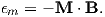(4.1)

ϵm is at a minimum when the magnetization M is aligned with the field B. Single-domain particles have a quasi-uniform magnetization and the application of a magnetic field does not change the net magnetization, which remains at saturation (Ms). The direction of all the magnetic spins could swing coherently toward the applied field. Yet the magnetizations in many particles do not rotate freely toward the magnetic field (or we would not have paleomagnetism!). There is another contribution to the energy of the magnetic particle associated with the magnetic crystal itself. This energy depends on the direction of magnetization in the crystal – it is anisotropic – and is called anisotropy energy. Anisotropy energy creates barriers to free rotation of the magnetization within the magnetic crystal, which lead to energetically preferred directions for the magnetization within individual single-domain grains.

There are many causes of anisotropy energy. The most important ones derive from the details of crystal structure (magnetocrystalline anisotropy energy), the state of stress within the particle (magnetostriction), and the shape of the particle, (shape anisotropy). We will consider these briefly in the following subsections.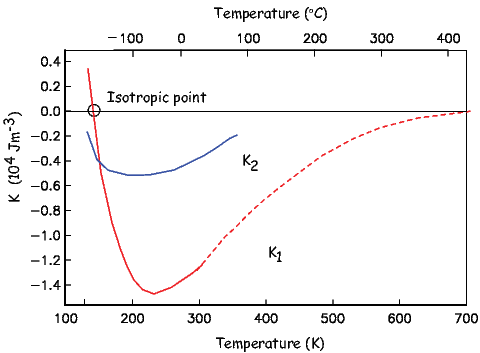Figure 4.2: Variation of K1 and K2 of magnetite as a function of temperature. Solid lines are data from Syono and Ishikawa (1963). Dashed lines are data from Fletcher and O’Reilly (1974).

#### 4.1.3 Magnetocrystalline anisotropy energy

For equant single-domain particles or particles with low saturation magnetizations, the crystal structure dominates the magnetic energy. In such cases, the so-called easy directions of magnetization are crystallographic directions along which magnetocrystalline energy is at a minimum. The energy surface shown in Figure 4.1c represents the magnetocrystalline anisotropy energy density, ϵa for magnetite at room temperature. The highest energy bulges are in directions perpendicular to the cubic faces ([001, 010, 100]). The lowest energy dimples are along the body diagonals (). Magnetite (above about 120K) has a cubic structure with direction cosines α123. These direction cosines are the angles between a given direction and the crystallographic axes [100, 010, 001] – see Appendix A.3.5 for review of direction cosines). For such a crystal the magnetocrystalline anisotropy energy density is given by: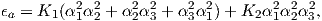(4.2)

where K1 and K2 are empirically determined magnetocrystalline anisotropy constants. In the case of (room temperature) magnetite, K1 is -1.35 x 104 Jm-3. Note that the units of the Ki are in Jm-3, so ϵa is in units of energy per unit volume (an energy density). If you work through the magnetocrystalline equation, you will find ϵa is zero parallel to the  axis, K14 parallel to the  and K13 + K227 parallel to the  direction (the body diagonal). So when K1 is negative, the  direction (body diagonal) has the minimum energy. This is the reason that there is a dimple in the energy surface along that direction in Figure 4.1c.

As a consequence of the magnetocrystalline anisotropy energy, once the magnetization is aligned with an easy direction, work must be done to change it. In order to switch from one easy axis to another (e.g. from one direction along the body diagonal to the opposite), the magnetization has to traverse a path over an energy barrier which is the difference between the energy in the easy direction and that in the intervening hard direction. In the case of magnetite at room temperature, we have this energy barrier as ϵ-ϵ or to first order K13 - K14 = K112.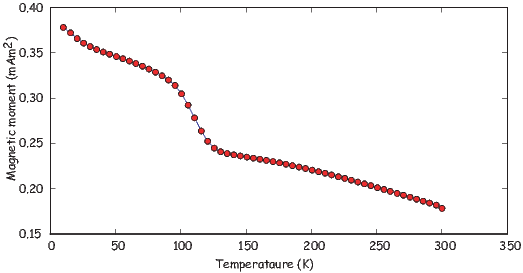Figure 4.3: Magnetization curve for magnetite as a function of temperature. The specimen was placed in a very large field, cooled to near absolute zero, then warmed up. The magnetization was measured as it warmed. When it goes through the Verwey transition (~110 K), a fraction of the magnetization is lost. Data downloaded from “w5000” in the Rock magnetic Bestiary collection at the Institute for Rock Magnetism.

Because electronic interactions depend heavily on inter atomic spacing, magnetocrystalline anisotropy constants are a strong function of temperature (see Figure 4.2). In magnetite, K1 changes sign at a temperature known as the isotropic point. At the isotropic point, there is no large magnetocrystalline anisotropy. The large energy barriers that act to keep the magnetizations parallel to the body diagonal are gone and the spins can wander more freely through the crystal. Below the isotropic point, the energy barriers rise again, but with a different topology in which the crystal axes are the energy minima and the body diagonals are the high energy states.

At room temperature, electrons hop freely between the ferrous and ferric ions on the B lattice sites, so there is no order. Below about 120 K, there is an ordered arrangement of the ferrous and ferric ions. Because of the difference in size between the two, the lattice of the unit cell becomes slightly distorted and becomes monoclinic instead of cubic. This transition occurs at what is is known as the Verwey temperature (Tv). Although the isotropic point (measured magnetically) and the Verwey transition (measured electrically) are separated in temperature by about 15o, they are related phenomena (the ordering and electron hopping cause the change in K1).

The change in magnetocrystalline anisotropy at low temperature can have a profound effect on the magnetization. In Figure 4.3 we show a typical (de)magnetization curve for magnetite taken from the “Rock magnetic bestiary” web site maintained at the Institute for Rock Magnetism: http://irm.umn.edu/bestiary. There is a loss of magnetization at around 100 K. This loss is the basis for low-temperature demagnetization (LTD). However, some portion of the magnetization always remains after low temperature cycling (called the low temperature memory), so the general utility of LTD may be limited.

Cubic symmetry (as in the case of magnetite) is just one of many types of crystal symmetries. One other very important form is the uniaxial symmetry which can arise from crystal shape or structure. The energy density for uniaxial magnetic anisotropy is: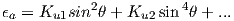(4.3)

Here the magnetocrystalline constants have been designated Ku1,Ku2 to distinguish them from K1,K2 used before. In this equation, when the largest uniaxial anisotropy constant, Ku1, is negative, the magnetization is constrained to lie perpendicular to the axis of symmetry. When Ku1 > 0, the magnetization lies parallel to it.

An example of a mineral dominated by uniaxial symmetry is hematite, a mineral with hexagonal crystal symmetry. The magnetization of hematite is quite complicated, as we shall learn in Chapters 6 and 7, but one source is magnetization is spin-canting (see Chapter 3) within the basal plane of the hexagonal crystal. Within the basal plane, the anisotropy constant is very low and the magnetization wanders fairly freely. However, the anisotropy energy away from the basal plane is strong, so the magnetization is constrained to lie within the basal plane.

#### 4.1.4 Magnetostriction - stress anisotropy

Exchange energy depends strongly on the details of the physical interaction between orbitals in neighboring atoms with respect to one another, hence changing the positions of these atoms will affect that interaction. Put another way, straining a crystal will alter its magnetic behavior. Similarly, changes in the magnetization can change the shape of the crystal by altering the shapes of the orbitals. This is the phenomenon of magnetostriction. The magnetic energy density caused by the application of stress to a crystal be approximated by: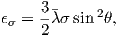where λ is an experimentally derived constant, σ is the stress, and θ is the angle of the stress with with respect to the c crystallographic axis. Moskowitz (1993b) measured the magnetostriction constants parallel to  and  in magnetite and found λ111 and λ100 to be 78.2x10-6 and -21.8x10-6 respectively. λ is given by: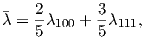so λ for magnetite is about 38 x 10-6. Stress has units of Nm-2 which have the same fundamental units as Jm-3, so λ is dimensionless. Note the similarity in form of magnetostriction and uniaxial anisotropy giving rise to a single “easy axis” within the crystal.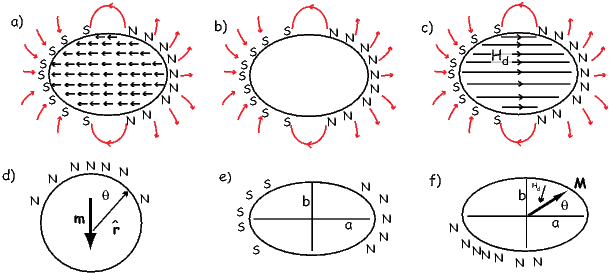Figure 4.4: a) Internal magnetizations within a ferromagnetic crystal. b) Generation of an identical external field from a series of surface monopoles. c) The internal “demagnetizing” field resulting from the surface monopoles. [Redrawn from O’Reilly, 1984]. d) Surface monopoles on a sphere. e) Surface monopoles on an ellipse, with the magnetization parallel to the elongation. f) Demagnetizing field Hd resulting from magnetization M at angle θ from a axis in prolate ellipsoid.

#### 4.1.5 Magnetostatic (shape) anisotropy

There is one more important source of magnetic anisotropy: shape. To understand how crystal shape controls magnetic energy, we need to understand the concept of the internal demagnetizing field of a magnetized body. In Figure 4.4a we show the magnetic vectors within a ferromagnetic crystal. These produce a magnetic field external to the crystal that is proportional to the magnetic moment (see Chapter 1). This external field is identical to a field produced by a set of free poles distributed over the surface of the crystal (Figure 4.4b). The surface poles do not just produce the external field, they also produce an internal field shown in Figure 4.4c. The internal field is known as the demagnetizing field (Hd). Hd is proportional to the magnetization of the body and is sensitive to the shape. For a simple sphere in Figure 4.4a and applied field condition shown in Figure 4.4d, the demagnetizing field is given by: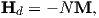where N is a demagnetizing factor determined by the shape. In fact, the demagnetizing factor depends on the orientation of M within the crystal and therefore is a tensor (see Appendix A.3.5 for review of tensors). The more general equation is Hd = N M where Hd and M are vectors and N is a 3 x 3 tensor. For now, we will simplify things by considering the isotropic case of a sphere in which N reduces to the single value scalar quantity N.

For a sphere, the surface poles are distributed over the surface such that there are none at the “equator” and most at the “pole” (see Figure 4.4d). Potential field theory shows that the external field of a uniformly magnetized body is identical to that of a centered dipole moment of magnitude m = vM (where v is volume). At the equator of the sphere as elsewhere, Hd = -NM. But the external field at the equator is equal to the demagnetizing field just inside the body because the field is continuous across the body. We can find the equatorial (tangential) demagnetizing field at the equator by substituting in the equatorial colatitude θ = 90 into Hθ in Equation 1.8 from Chapter 1), so: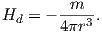Using the fact that magnetization (in units of Am-1) is the moment (in units of Am2) per unit volume (in units of m3) and the volume of a sphere is 4 3πr3, we have: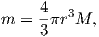so substituting and solving for Hd we get Hd = -1 3M, hence N = 1 3.

Different directions within a non-spherical crystal will have different distributions of free poles (see Figures 4.4e,f). In fact the surface density of free poles is given by σm = M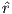. Because the surface pole density depends on the direction of magnetization, so too will N. In the case of a prolate ellipsoid magnetized parallel to the elongation axis a (Figure 4.4e), the free poles are farther apart than across the grain, hence, intuitively, the demagnetizing field, which depends on 1∕r2, must be less than in the case of a sphere. Thus, Na <1 3. Similarly, if the ellipsoid is magnetized along b (Figure 4.4e), the demagnetizing field is stronger or Nb > 1 3.

Getting back to the magnetostatic energy density, ϵm = MB, remember that B includes both the external field Be = -μoHe and the internal demagnetizing field μoN M. Therefore, magnetostatic energy density from both the external and internal fields is given by: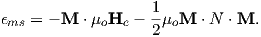(4.4)

The two terms in Equation 4.4 are the by now familiar magnetostatic energy density ϵm, and the magnetostatic self energy density or the demagnetizing energy density ϵd. ϵd can be estimated by “building” a magnetic particle and considering the potential energy gained by each incremental volume dv as it is brought in (-μoMdv Hd) and integrating. The 1 2 appears in order to avoid counting each volume element twice and the v disappears because all the energies we have been discussing are energy densities – the energy per unit volume.

For the case of a uniformly magnetized sphere, we get back to the relation Hd = -NM and ϵd simplifies to: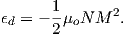(4.5)

In the more general case of a prolate ellipsoid, M can be represented by the two components parallel to the a and b axes (see Figure 4.4f) with unit vectors parallel to them ,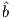. So, M = M cosθ + M sinθ. Each component of M has an associated demagnetizing field Hd = -NaM cosθ - NbM sinθ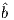where Na,Nb are the eigenvalues of the tensor N (the values of the demagnetizing tensor along the principal axes a and b). In this case, the demagnetizing energy can be written as: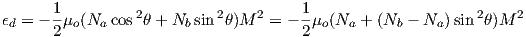(4.6)

In an ellipsoid with three unequal axes a,b,c, Na + Nb + Nc = 1 (in SI; in cgs units the sum is 4π). For a long needle-like particle, Na 0 and Nb = Nc 1 2. A useful approximation for nearly spherical particles is Na = 1 3[1 - 2 5(2 - b a - c a)] (Stacey and Banerjee, 1974). For more spheroids, see Nagata (1961, p. 70) and for the general case, see Dunlop and �zdemir (1997). In the absence of an external field, the magnetization will be parallel to the long axis (θ = 0) and the magnetostatic energy density (also known as the ‘self’ energy is given by: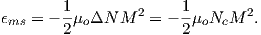(4.7)

Note that the demagnetizing energy in Equation 4.6 has a uniaxial form, directionally dependent only on θ, with the constant of uniaxial anisotropy Ku = 1 2ΔoM2. ΔN is the difference between the largest and smallest values of the demagnetizing tensor Nc - Na.

For a prolate ellipsoid Nc = Nb and choosing for example c∕a = 1.5 we find that Na -Nc =~ 0.16. The magnetization of magnetite is 480 kAm-1, so Ku 2.7 x 104 Jm-3. This is somewhat larger than the absolute value of K1 for magnetocrystalline anisotropy in magnetite (K1= -1.35 x 104 Jm-3), so the magnetization for even slightly elongate grains will be dominated by uniaxial anistropy controlled by shape. Minerals with low saturation magnetizations (like hematite) will not be prone to shape dominated magnetic anisotropy, however.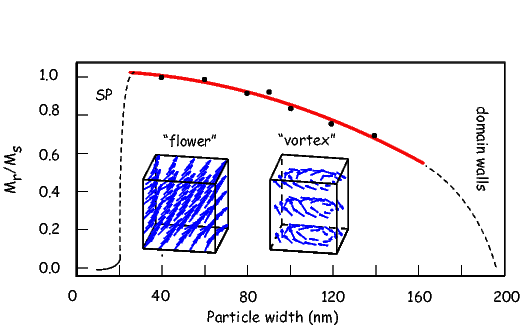Figure 4.5: Possible non-uniform magnetization configurations that reduce self energy for magnetite with increasing particle widths. The net remanent magnetization reduces with increasingly non-uniform spin configurations. [Data from Tauxe et al., 2002.]

#### 4.1.6 Magnetic energy and magnetic stability

Paleomagnetists worry about how long a magnetization can remain fixed within a particle and we will begin to discuss this issue later in the chapter. It is worth pointing out here that any discussion of magnetic stability will involve magnetic anisotropy energy because this controls the energy required to change a magnetic moment from one easy axis to another. One way to accomplish this change is to apply a magnetic field sufficiently large that its magnetic energy exceeds the anisotropy energy. The magnetic field capable of flipping the magnetization of an individual uniformly magnetized particle (at saturation, or Ms) over the magnetic anisotropy energy barrier is the microscopic coercivity Hk. For uniaxial anisotropy (K = Ku) and for cubic magnetocrystalline anisotropy (K = K1), microscopic coercivity is given by: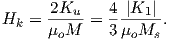(4.8)

respectively (see Dunlop and �zdemir, 1997 for a more complete derivation). For elongate particles dominated by shape anisotropy, Hk reduces to ΔNM. [Note that the units for coercivity as derived here are in Am-1, although they are often measured using instruments calibrated in tesla. Technically, because the field doing the flipping is inside the magnetic particle and B (measured in tesla) depends on the magnetization M as well as the field H (Equation 1.4), coercivity should be written as μoHk if the units are quoted in tesla. Microscopic coercivity is another parameter with many names: flipping field, switching field, intrinsic coercivity and also more loosely, the coercive field and coercivity. We will come back to the topic of coercivity in Chapter 5. ]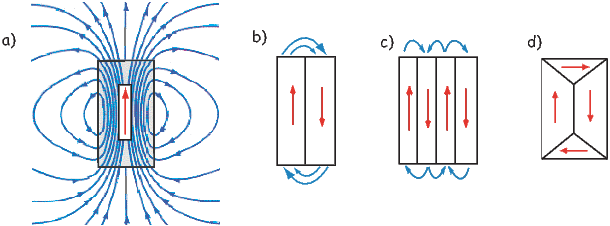Figure 4.6: A variety of domain structures of a given particle. a) Uniformly magnetized (single domain). [Adapted from Tipler, 1999.] b) Two domains. c) Four domains in a lamellar pattern. d) Essentially two domains with two closure domains.

### 4.2 Magnetic domains

So far we have been discussing hypothetical magnetic particles that are uniformly magnetized. Particles with strong magnetizations (like magnetite) have self energies that quickly become quite large because of the dependence on the square of the magnetization. We have been learning about several mechanisms that tend to align magnetic spins. In fact in very small particles of magnetite (< 40 nm), the spins are essentially lined up. The particle is uniformly magnetized and we called it single domain (SD). In larger particles (~80 nm) the self energy exceeds the other exchange and magnetocrystalline energies and crystals have distinctly non-uniform states of magnetization.

There are many strategies possible for magnetic particles to reduce self energy. Numerical models (called micromagnetic models) can find internal magnetization configurations that minimize the energies discussed in the preceding sections. Micromagnetic simulations for magnetite particles (e.g. Schabes and Bertram, 1988) allow us to peer into the state of magnetization inside magnetic particles. These simulations give a picture of increasing complexity from so-called flower to vortex (Figure 4.5) remanent states. These particles share many properties of the uniformly magnetized single domain particles and are called pseudo-single domain (PSD) particles.

As particles grow larger (>~200 nm), they break into multiple magnetic domains, separated by narrow zones of rapidly changing spin directions called domain walls. Magnetic domains can take many forms. We illustrate a few in Figure 4.6. The uniform case (single domain) is shown in Figure 4.6a. The external field is very large because the free poles are far apart (at opposite ends of the particle). When the particle organizes itself into two domains (Figure 4.6b), the external field is reduced by about a factor of two. In the case of four lamellar domains (Figure 4.6c), the external field is quite small. The introduction of closure domains as in Figure 4.6d reduces the external field to nothing.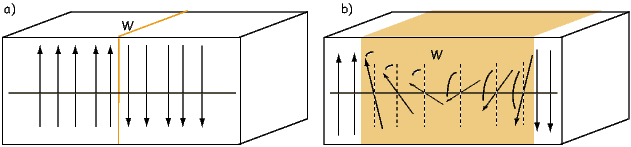Figure 4.7: Examples of possible domain walls. a) There is a 180 switch from one atom to the next. The domain wall is very thin, but the exchange price is very high. b) There is a more gradual switch from one direction to the other [note: each arrow represents several 10’s of unit cells]. The exchange energy price is lower, but there are more spins in unfavorable directions from a magnetocrystalline point of view.

As you might already suspect, domain walls are not “free”, energetically speaking. If, as in Figure 4.7a, the spins simply switch from one orientation to the other abruptly, the exchange energy cost would be very high. One way to get around this to spread the change over several hundred atoms, as sketched in Figure 4.7b. The wall width δ is wider and the exchange energy price is much less. However, there are now spins in unfavorable directions from a magnetocrystalline point of view (they are in “hard” directions). Exchange energy therefore favors wider domain walls while magnetocrystalline anisotropy favors thin walls. With some work (see e.g., Dunlop and �zdemir, 1997, pp. 117-118), it is possible to come up with the following analytical expressions for wall width (δw) and wall energy density (ϵw):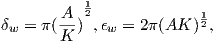(4.9)

where A is the exchange constant (see Section 4.1.1) and K is the magnetic anisotropy constant (e.g., Ku or K1). Note that ϵw is the energy density per unit wall area, not per volume. Plugging in values for magnetite given previously we get δw = 90 nm and ϵw = 3x 10-3Jm-2.

In Figure 4.8 we plot the self energy (Equation 4.12) and the wall energy (ϵw from Equation 4.9) for spheres of magnetite. We see that the wall energy in particles with diameters of some 50 nm is less than the self energy, yet the width of the walls about twice as wide as that. So the smallest wall is really more like the vortex state and it is only for particles larger than a few tenths of a micron that true domains separated by discrete walls can form. Interestingly, this is precisely what is predicted from micromagnetic modelling (e.g., Figure 4.5).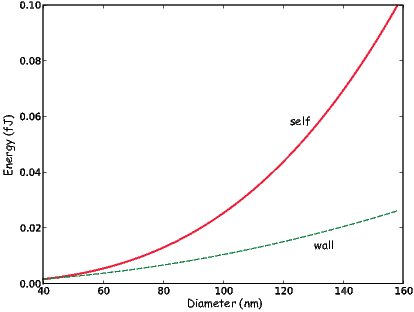Figure 4.8: Comparison of “self” energy versus the energy of the domain wall in magnetite spheres as a function of particle size.

How can we test the theoretical predictions of domain theory? Do domains really exist? Are they the size and shape we expect? Are there as many as we would expect? In order to address these questions we require a way of imaging magnetic domains. Bitter (1931) devised a way for doing just that. Magnetic domain walls are regions with large stray fields (as opposed to domains in which the spins are usually parallel to the sides of the crystals to minimize stray fields). In the Bitter technique magnetic colloid material is drawn to the regions of high field gradients on highly polished sections allowing the domain walls to be observed (see Figure 4.9a).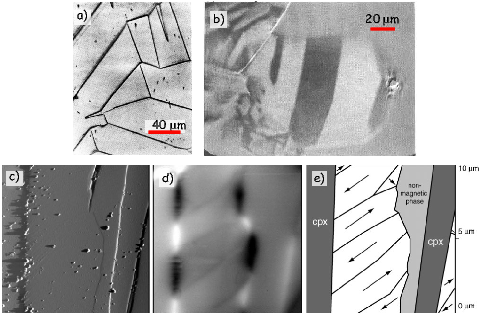Figure 4.9: a) Bitter patterns from an oriented polished section of magnetite. [Figure from �zdemir et al., 1995]. b) Domains revealed by longitudinal magneto-optical Kerr effect. [Image from Heider and Hoffmann, 1992.] c-e) Magnetic force microscopy technique. [Images from Feinberg et al., 2005.] c) Image of topography of surface of a magnetite inclusion in a non-magnetic matrix. d) Magnetic image from MFM techqnique. e) Interpretation of magnetizations of magnetic domains.

There are by now other ways of imaging magnetic domains. We will not review them all here, but will just highlight the ways that are more commonly used in rock and paleomagnetism. The magneto-optical Kerr effect or MOKE uses the interaction between polarized light and the surface magnetic field of the target. The light interacts with the magnetic field of the sample which causes a small change in the light’s polarization and ellipticity. The changes are detected by reflecting the light into nearly-crossed polarizers. The longitudinal Kerr effect can show the alignment of magnetic moments in the surface plane of the sample. Domains with different magnetization directions show up as lighter or darker regions in the MOKE image (see Figure 4.9b.)

Another common method for imaging magnetic domains employs a technique known as magnetic force microscopy. Magnetic force microscopy (MFM) uses a scanning probe microscope that maps out the vertical component of the magnetic fields produced by a highly polished section. The measurements are made with a cantilevered magnetic tip that responds to the magnetic field of the sample. In practice, the measurements are made in two passes. The first establishes the topography of the sample (Figure 4.9c). Then in the second pass, the tip is raised slightly above the surface and by subtracting the topographic only signal the attraction of the magnetic surface can be mapped (Figure 4.9d). Figure 4.9e shows an interpretation of the magnetic directions of different magnetic domains.

### 4.3 Thermal energy

We have gone some way toward answering the questions posed at the beginning of the chapter. We see now that anisotropy energy, with contributions from crystal structure, shape and stress, that inhibits changes in the magnetic direction thereby offering a possible mechanism whereby a given magnetization could be preserved for posterity. We also asked the question of what allows the magnetization to come into equilibrium with the applied magnetic field in the first place; this question requires a little more work to answer. The key to this question is to find some mechanism which allows the moments to “jump over” magnetic anisotropy energy barriers. One such mechanism is thermal energy ET , which was given in Chapter 3 as: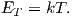We know from statistical mechanics that the probability P of finding a grain with a given thermal energy sufficient to overcome some anisotropy energy Ea and change from one easy axis to another is P = exp(-Ea∕ET ). Depending on the temperature, such grains may be quite rare, and we may have to wait some time t for a particle to work itself up to jumping over the energy barrier.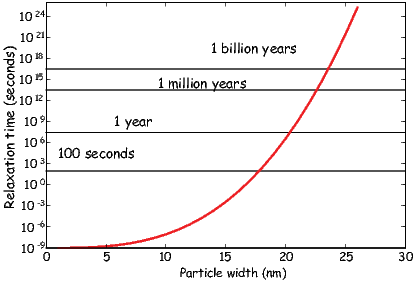Figure 4.10: Relaxation time in magnetite ellipsoids as a function of grain width in nanometers (all length to width ratios of 1.3:1.)

Imagine a block of material containing a random assemblage of magnetic particles that are for simplicity uniformly magnetized and dominated by uniaxial anisotropy. Suppose that this block has some initial magnetization Mo and is placed in an environment with no ambient magnetic field. Anisotropy energy will tend to keep each tiny magnetic moment in its original direction and the magnetization will not change over time. At some temperature, certain grains will have sufficient energy to overcome the anisotropy energy and flip their moments to the other easy axis. As the energy surface is spherical, with no dimples or protruberances, there is no preferred direction and, over time, the magnetic moments will become random. Therefore, the magnetization as a function of time in this simple scenario will decay to zero. The equation governing this decay is: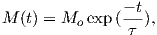(4.10)

where t is time and τ is an empirical constant called the relaxation time. Relaxation time is the time required for the remanence to decay to 1∕e of Mo. This equation is the essence of what is called N�el theory (see, e.g., N�el, 1955). The value of τ depends on the competition between magnetic anisotropy energy and thermal energy. It is a measure of the probability that a grain will have sufficient thermal energy to overcome the anisotropy energy and switch its moment. Therefore in zero external field: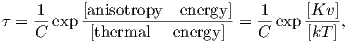(4.11)

where C is a frequency factor with a value of something like 1010 s-1. The anisotropy energy is given by the dominant anisotropy parameter K (either Ku,K1, or λ) times the grain volume v.

Thus, the relaxation time is proportional to anisotropy constant and volume, and is inversely related to temperature. Relaxation time τ varies rapidly with small changes in v and T. To see how this works, we can take Ku for slightly elongate cuboids of magnetite (length to width ratio of 1.3 to 1) and evaluate relaxation time as a function of particle width (see Figure 4.10). There is a sharp transition between grains with virtually no stability (τ is on the order of seconds) and grains with stabilities of billions of years.

Grains with τ 102 - 103 seconds have sufficient thermal energy to overcome the anisotropy energy frequently and are unstable on a laboratory time scale. In zero field, these grain moments will tend to rapidly become random, and in an applied field, also tend to align rapidly with the field. The net magnetization is related to the field by a Langevin function (see Section 3.2.2 in Chapter 3). Therefore, this behavior is quite similar to paramagnetism, hence these grains are called superparamagnetic (SP). Such grains can be distinguished from paramagnets, however, because the field required to saturate the moments is typically much less than a tesla, whereas that for paramagnets can exceed hundreds of tesla.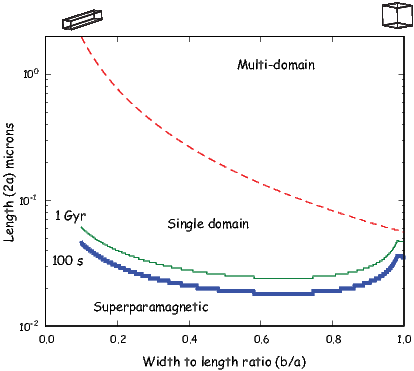Figure 4.11: Expected domain states for various sizes and shapes of parallelopipeds of magnetite at room temperature. The parameters a and b are as in Figure 4.4e. Heavy blue (thin green) line is the superparamagnetic threshold assuming a relaxation time of 100s (1 Gyr). Dashed red line marks the SD/MD threshold size. Calculations done using assumptions and parameters described in the text.

### 4.4 Putting it all together

We are now in a position to pull together all the threads we have considered in this chapter and make a plot of what sort of magnetic particles behave as superparamagnets, which should be single domain and which should be multi-domain according to our simple theories. We can estimate the superparamagnetic to single domain threshold for magnetite as a function of particle shape by finding for the length (2a) that gives a relaxation time of 100 seconds as a function of width to length ratio (b∕a) for parallelopipeds of magnetite (heavy blue line in Figure 4.11). To do this, we follow the logic of Evans and McElhinny (1969) and Butler and Banerjee (1975). In this Evans diagram, we estimated relaxation time using Equation 4.11, plugging in values of K as either the magnetocrystalline effective anisotropy constant ( 1_ 12K1) or the shape anisotropy constant (1 2ΔoM2), whichever was less. We also show the curve at which relaxation time is equal to 1 Gyr, reinforcing the point that very small changes in crystal size and shape make profound differences in relaxation time. The figure also predicts the boundary between the single domain field and the two domain field, when the energy of a domain wall is less than the self energy of a particle that is uniformly magnetized. This can be done by evaluating wall energy with Equation 4.9 for a wall along the length of a parallelopiped and area (4ab) as compared to the self energy (1 2μoNaM2v) for a given length and width to length ratio. When the wall energy is less than the self energy, we are in the two domain field.

Figure 4.11 suggests that there is virtually no SD stability field for equant magnetite; particles are either SP or MD (multi-domain). As the width to length decreases (the particle gets longer), the stability field for SD magnetite expands. Of course micromagnetic modelling shows that there are several transitional states between uniform magnetization (SD) and MD, i.e. the flower and vortex remaent states (see Fabian et al., 1996), but Figure 4.11 has enormous predictive power and the version of Butler and Banerjee (1975), (which is slightly different in detail) continues to be used extensively. It is worth pointing out however, that the size at which domain walls appear in magnetite is poorly constrained because it depends critically on the exact shape of the particle, its state of stress and even its history of exposure to past fields. Estimates in the literature range from as small as 20 nm to much larger (up to 100 nm) depending on how the estimates are made. Nonetheless, it is probably true that truly single domain magnetite is quite rare in nature, yet more complicated states are difficult to treat theoretically. Therefore most paleomagnetic studies rely on predictions made for single domain particles.

SUPPLEMENTAL READING: Dunlop and �zdemir (1997), Chapters 2.8 and 5.

### 4.5 Problems

Problem 1

Assume that the magnetization of magnetite is about 4.8 x 105 Am-1. Using values for other parameters from the text, write a Python program to calculate the following:

a) Self energy (or magnetostatic energy) for a sphere 1, 10 and 100 μm in diameter. [Hint: see Equation 4.12 below for the ‘self’ energy density. Also, remember the difference between energy and energy density!]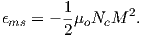(4.12)

b) Magnetostatic (shape) anisotropy energy for an ellipsoid whose principal semi-axis is 1 μm and whose major and minor semi-axes are each 0.25 μm. You may use the “nearly spherical” approximation in the text.

c) The critical radius of a sphere at which wall energy equals self energy.

Problem 2

Calculate grain diameter for magnetite spheres with τs of 10-1, 10, 102, 103, 105, 109, 1015 seconds. Use values for Boltzmann’s constant, C (the frequency factor) and |K1| at room temperature (300K).

Problem 3 [From Jeff Gee]

a) Consider a highly elongate rod (needle-shaped grain) of magnetite. Explain why the demagnetizing factor along the long axis of the rod is about zero while that across the long axis is about one half.

b) The file Chapter_4/prolate.txt gives the values of demagnetizing factors for a prolate ellipsoid (with axes a> b=c). For an elongate rod of magnetite with range of aspect ratios (AR = c:b) provided in the table, plot the magnetostatic self energy density in the absence of an external field. Use this plot to estimate the aspect ratio at which shape anisotropy will be equal that of magnetocrystalline anisotropy (use a value of K1 at room temperature (300K) of -1.43 x 104 J/m3).

c) What is the maximum microscopic coercivity (Hk) for such an elongate grain of magnetite (assume an infinitely long grain)? Coercivities are more commonly reported in units of T so provide this corresponding value as well.